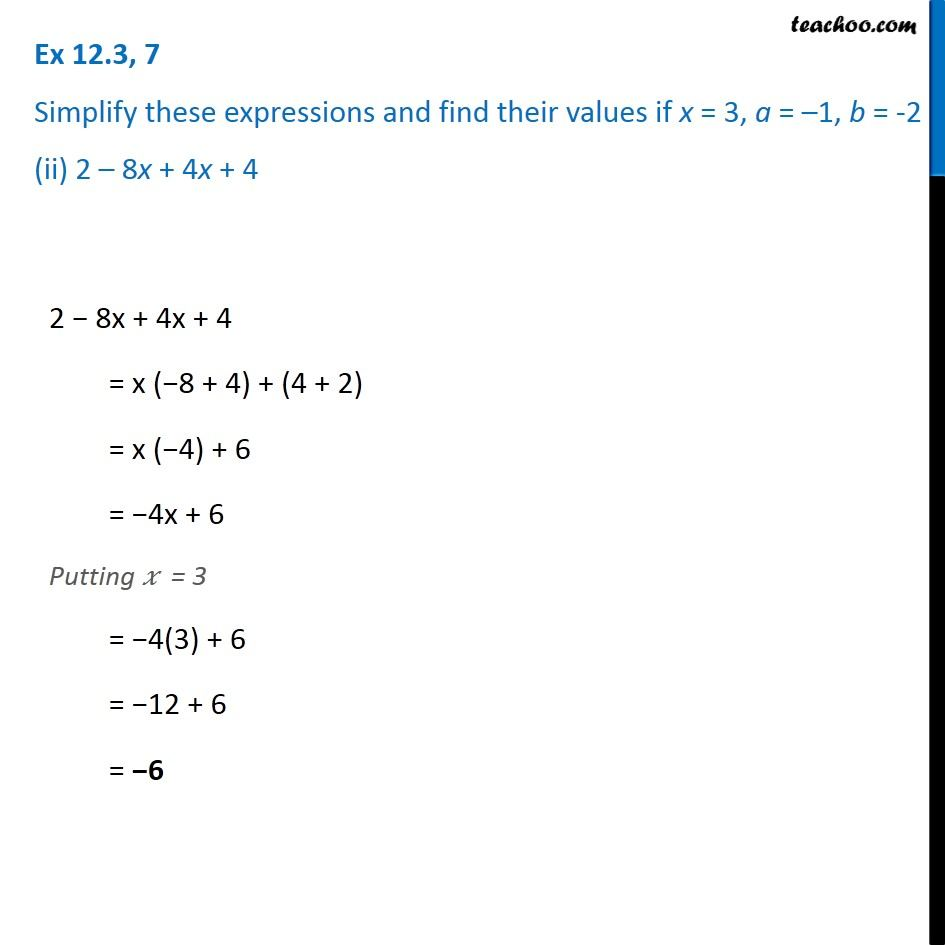Ex 12.3

Chapter 12 Class 7 Algebraic Expressions
Serial order wiseGet live Maths 1-on-1 Classs - Class 6 to 12

### Transcript

Ex 12.3, 7 Simplify these expressions and find their values if x = 3, a = –1, b = -2 (ii) 2 – 8x + 4x + 4 2 − 8x + 4x + 4 = x (−8 + 4) + (4 + 2) = x (−4) + 6 = −4x + 6 Putting 𝑥 = 3 = −4(3) + 6 = −12 + 6 = −6